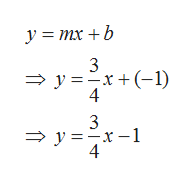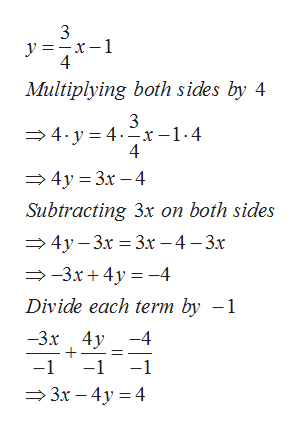# Write an equation in the form Ax + By = C, given that m=3/4 and y-intercept is (0,-4), where A, B and C are integers.

Question
30 views

Write an equation in the form Ax + By = C, given that m=3/4 and y-intercept is (0,-4), where A, B and C are integers.

check_circle

Step 1

First we will substitute the value of slope m= 3/4 and y-intercept b=-1 in slope-intercept form y= mx+b.help_outlineImage Transcriptioncloseу%3D тх + b 3 — у %3D—х +(-1) 4 3 — у %3D—х —1 4 fullscreen
Step 2

In order to get rid fraction 3/4, we need to multiply each term by 4.

Multiplying both sides by 4, we get 4y=3x-4.

Now, subtracting 3x on ...help_outlineImage Transcriptionclose3 4 Multiplying both sides by 4 3 4.y 4.x-1-4 4 4y 3x -4 Subtracting 3x on both sides 4y 3r 3x - 4 -3x 3.x 4y-4 Divide each term by -1 -3х 4у -4 -1 -1 -1 3x - 4y 4 fullscreen

### Want to see the full answer?

See Solution

#### Want to see this answer and more?

Solutions are written by subject experts who are available 24/7. Questions are typically answered within 1 hour.*

See Solution
*Response times may vary by subject and question.
Tagged in

### Algebra